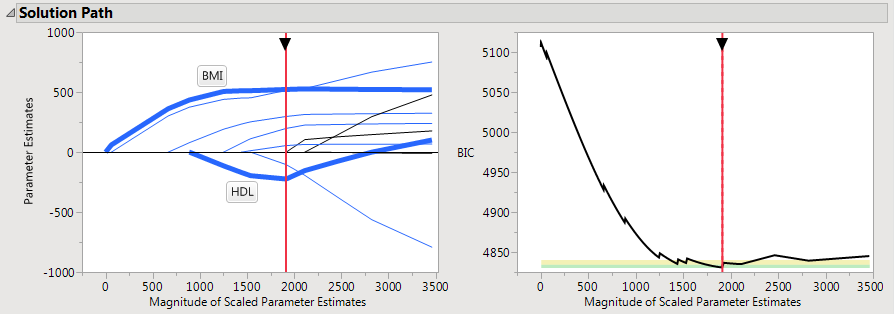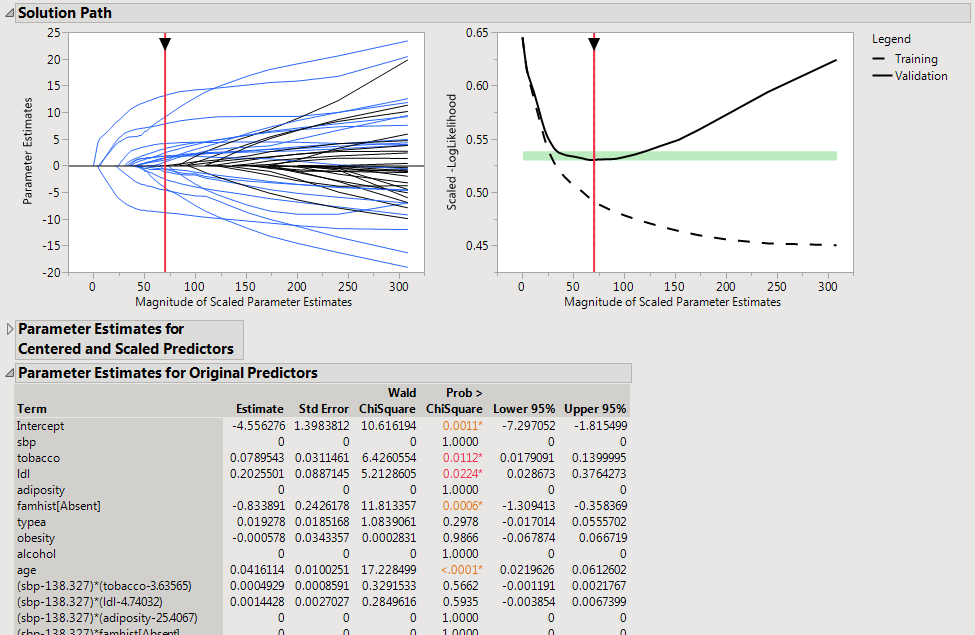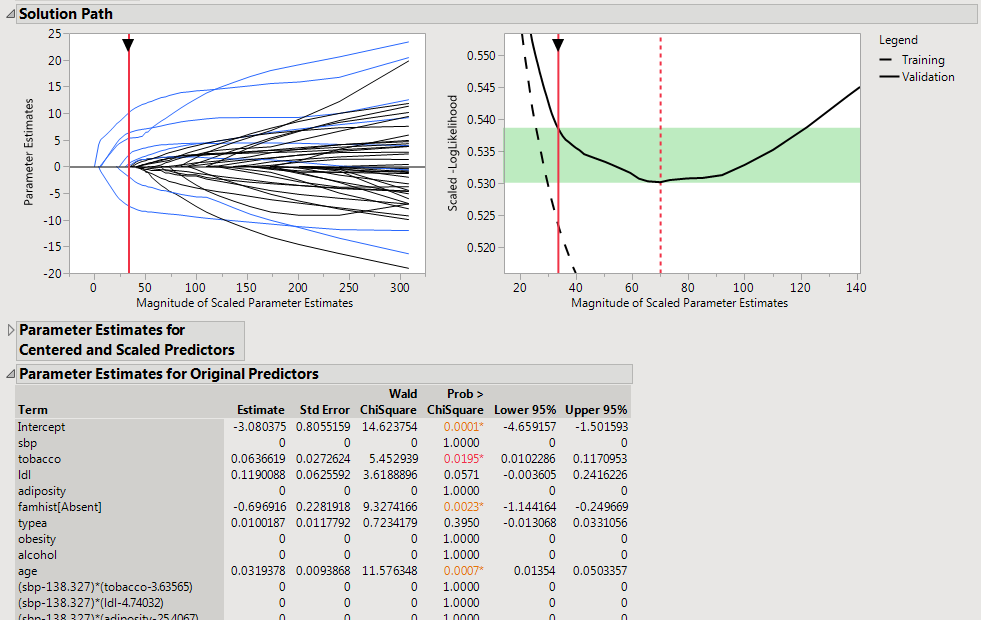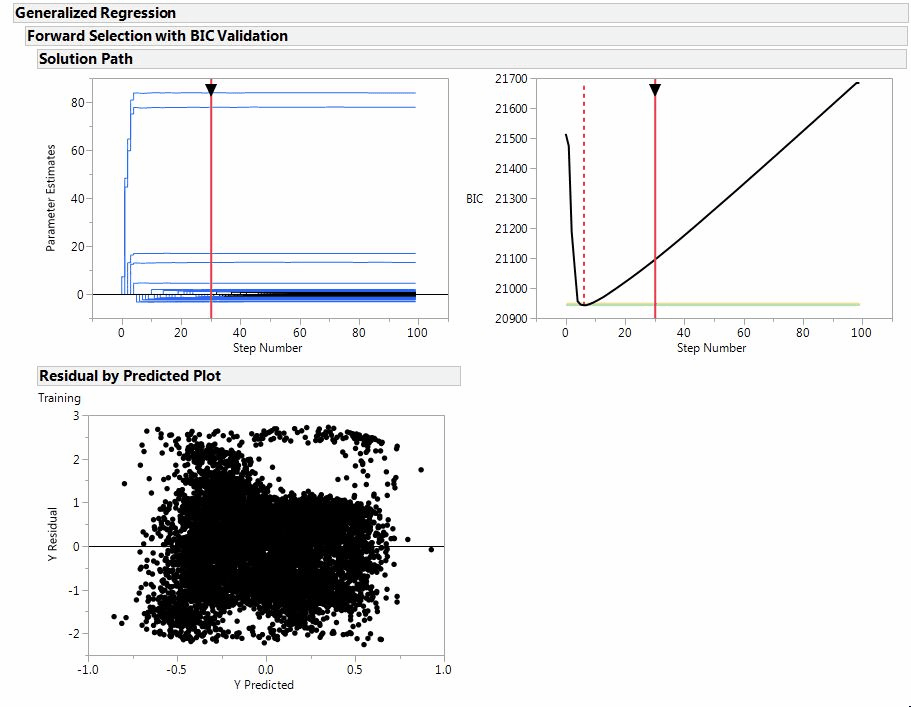Choose Language Hide Translation Bar
Coming in JMP Pro 12: Interactive model building

The Generalized Regression platform was introduced in JMP Pro 11 for fitting penalized regression models. Our focus for JMP Pro 12 has been to make model building an easy and natural process using the Generalized Regression platform (we like to call it Genreg for short). This post will focus on the new feature that I am most excited about in Genreg: the interactive solution path.

As noted in previous posts, a penalized regression fit does not result in a single regression model. Instead, we end up with a sequence of candidate models from which we choose the best fitting model based on a validation method (like cross-validation). The best way to summarize the sequence of candidate models is to plot the solution path as in Figure 1, a lasso fit of the diabetes data in the sample data folder in JMP.Figure 1: Lasso solution path for the diabetes data

On the left side of Figure 1, we see a summary of how each variable enters the model and changes as a function of the lasso penalty. I have labeled two of the paths and made them bold for emphasis. Here BMI (body mass index) is the first variable to enter the model for predicting the progression of diabetes. As the lasso penalty is relaxed (moving from left to right in the graph), the coefficient for BMI steadily increases until it levels off around 500. HDL (the good cholesterol) is the fourth variable to enter our model. It enters the model with a negative coefficient but actually has a positive coefficient by the end of the path. This sign change reminds us why variable selection is so important: Choosing one model instead of another can mean the difference between concluding that HDL cholesterol speeds up, slows down or has no impact on diabetes progression. The best model (based on the Bayesian Information Criterion) is marked by the vertical red line.

On the right side of Figure 1, we see how well the candidate models fit the data as a function of the penalty. Here, we are using the BIC for validation (smaller is better), but the results for cross-validation can be summarized in the same way. As we relax the penalty (moving left to right), the model improves to a point, and then it starts to get worse/overfit. Once again, we mark the best solution using a vertical red line.

So what is special about the solution path in JMP Pro 12? It's interactive! That means that we can click on the vertical line in Figure 1 and drag it to explore all of the candidate models in the solution path. As we use the handle in the solution path to change the model, everything in the report is updated to reflect the new model: parameter estimates, residual plots, Profilers and so on. This allows us to quickly explore candidate models that are not necessarily the best-fitting, but are still interesting or useful. For example, maybe there is a much simpler model that performs nearly as well as the best. Now we can quickly locate that simpler model and use it. Alternatively, there are situations where we would want to drag the handle to the right and use a larger model that performs similarly to the best. By using a larger model, we can feel more confident that we have identified the factors that truly are influencing the response variable.

Now let's look at an example of using the interactive solution path to build a logistic regression model for the South African heart disease data. Figure 2 shows the solution path and a portion of the parameter estimates table for a lasso fit tuned using 5-fold cross-validation. We can see that the validation likelihood flattens out around the best model, meaning that we have an opportunity to back up to a more parsimonious model that still fits very well. In fact, JMP even provides a green shaded zone where the performance of the models is similar to the best model.Figure 2: Best Model for the Heart Disease Data

In Figure 3, we have zoomed in on the right side of the solution path so that the range of models in the green zone are more obvious. We have also backed up to the smallest model inside the green zone. Notice that the parameter estimates have changed, and two of the interactions have dropped out of the model. Our new model has less than half as many non-zero terms as the best model, so it is substantially easier to interpret while still fitting very well.Figure 3: A Much Simpler Model for the Heart Disease Data

It helps to see the interactive solution path in action. Figure 4 is an animation of building a regression model for a particularly interesting data set (you may have to click on the figure to see the animation). For more information about creating unique data sets like what you see in Figure 4, check out the website of NC State University professor Leonard Stefanski.Figure 4: Interactive Solution Path in Action

We have added a variety of exciting new features to the Genreg platform, but I am most excited about the interactive solution path. The interactivity allows us to quickly and easily build regression models in JMP Pro. Some of the other highlights in Genreg include:

• Substantially improved computation times
• Forward Selection
• More distributions for modeling the response (Exponential, Beta, Beta-binomial, Cauchy, and more)
• Quantile regression
• Inverse prediction
• More diagnostic plots
• Article Tags